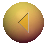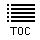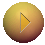Problems with Newton
Slide 3 of 22Go to slide:

Newton's laws of mechanics and universal gravitation worked wonderfully well in describing virtually all terrestial phenomena as well as the motions of the moon and the planets. In this sense Newton provided a beautiful synthesis of two previously distinct sets of phenomena: the terrestrial and the celestial. However, as with all theories and combination of experimental results and conceptual reasoning ultimately forced Newton's gravitation theory to be modified and replaced by Einsein's theory of general relativity. There were essentially three problems with Newton's theory. First of all, there was a conceptual problem. In Newtonian gravity, the strength of the gravitational force bewteen two bodies was proportional to the product of the inertial masses of the bodies. Inertial mass was therefore doing double duty: by definition, it was a measure of the resistence of an object to a change in velocity. In addition, inertial mass seem to also play a role as the "gravitational charge". In much the same way that electric charge determines the strength of electrostatic forces between two charged objects, the inertial mass (a.k.a. the gravitational charge) determines the strength of the corresponding gravitational force. This is the reason that, as found by Galileo, all objects fall to Earth at precisely the same rate. The reason for this double duty is a complete mystery in the context of Newtonian mechanics, but is essentially a trivial consequence of Einsteinian gravity. The second problem with Newton's theory was that it described gravity as an instantaneous force of attraction between two massive objects. Consequently, if you move one of them, the other knows about the move immediately due to the change in gravitation, irrespective of the distance between them. FInally, and most importantly, there was a discrepancy, albeit very tiny, between the predictions of Newton's theory, and experimental observation for the precession of Mercury's orbit.